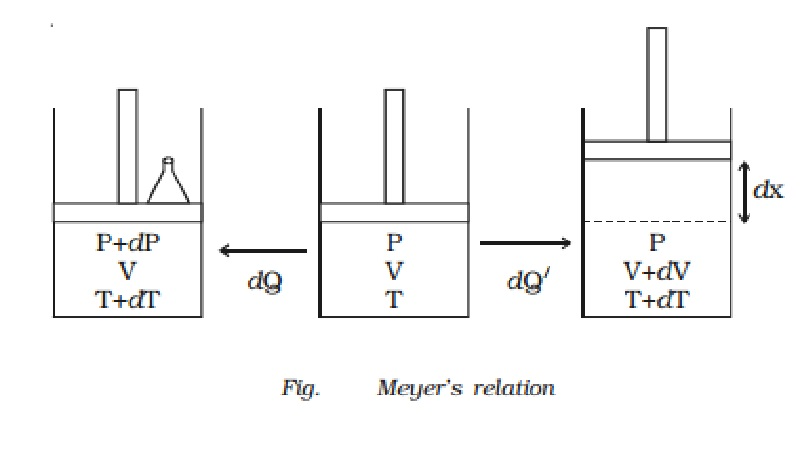Home | | Physics | | Physics | Relation between Cp and Cv (Meyer's relation)

# Relation between Cp and Cv (Meyer's relation)Let us consider one mole of an ideal gas enclosed in a cylinder provided with a frictionless piston of area A. Let P, V and T be the pressure, volume and absolute temperature of gas respectively (Fig.).

Relation between Cp and Cv (Meyer?s relation)

Let us consider one mole of an ideal gas enclosed in a cylinder provided with a frictionless piston of area A. Let P, V and T be the pressure, volume and absolute temperature of gas respectively (Fig.).A quantity of heat dQ is supplied to the gas. To keep the volume of the gas constant, a small weight is placed over the piston. The pressure and the temperature of the gas increase to P + dP and T + dT respectively. This heat energy dQ is used to increase the internal energy dU of the gas. But the gas does not do any work (dW = 0).

dQ = dU = 1 ? Cv ? dT ... (1)

The additional weight is now removed from the piston. The piston now moves upwards through a distance dx, such that the pressure of  the enclosed gas is equal to the atmospheric pressure P. The temperature of the gas decreases due to the expansion of the gas.

Now a quantity of heat dQ? is supplied to the gas till its temperature becomes T + dT. This heat energy is not only used to increase the internal energy dU of the gas but also to do external work dW in moving the piston upwards.

dQ?  = dU + dW

Since the expansion takes place at constant pressure,

dQ = CpdT

CpdT = CvdT + dW  ????(2)

Work done, dW = force ? distance

= P ? A ? dx

dW = P dV (since A ? dx = dV, change in volume)

CpdT = CvdT + P dV   ????(3)

The equation of state of an ideal gas is

PV = RT

Differentiating both the sides

PdV = RdT  ????(4)

Substituting equation (4) in (3),

CpdT = CvdT + RdT

Cp = Cv + R

Cp - Cv = R

This equation is known as Meyer?s relation

Study Material, Lecturing Notes, Assignment, Reference, Wiki description explanation, brief detail

Related Topics